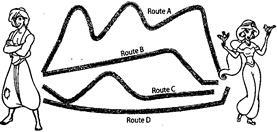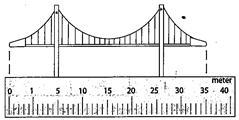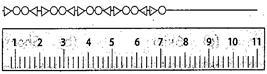Courses

Test: Measurement Of Length - 2

10 Questions MCQ Test Mathematics for Class 3: NCERT | Test: Measurement Of Length - 2

Description
Attempt Test: Measurement Of Length - 2 | 10 questions in 20 minutes | Mock test for Class 3 preparation | Free important questions MCQ to study Mathematics for Class 3: NCERT for Class 3 Exam | Download free PDF with solutions
QUESTION: 1

Alladin has to reach to Jasmine as soon as possible. He is looking for the shortest route so that he can reach on time. Can you tell him which the shortest route is?Solution:

Since, the shortest route is route b Therefore, the answer is option (d) Route D

QUESTION: 2

How long is the bridge?Solution:

Since, the bridge ends at 35 on the scale and length of the bridge is in meters. Therefore, the answer is option (c) 35 meters.

QUESTION: 3

How long is the necklace?Solution:

Since, the necklace ends at 7 on the scale and length of a necklace is measured in centimeters. Therefore, the answer is option (b) 7 centimeters.

QUESTION: 4

Direction: Read the passage given below and answer the questions that follow: Spiderman organized a long jump competition for the students of class 3. Many students took part in the competition. Everybody was very excited with the competition. The competition stated with the long jump made by Spiderman. Then he called Minku, Rinku. Tinku, Chinku, Pinku and D'nku to make their jumps. Minku started the game by jumping at a distance of 2 meter. Pinku's jump was 3 and half metre long. Then Tinku jumped at a distance of 250 centimetre while Chinku jumped at a distance of 300 centimetre. Dinku's jump was 3 meter long and Rinku's jump was 2 and half metre long. When the game ended Spiderman recorded the distances jumped by the children. Spiderman divided to give his mark to the winner. Who is the winner of the competition?

Solution:

The longest jump was made by Pinku i.e. 3 and half metre, so he is the winner. Therefore, the answer is option a Pinku.

QUESTION: 5

Direction: Read the passage given above and answer the questions that follow: Who jumped the least distance?

Solution:

The shortest jump was of 250 cm made by Tinku. Therefore, the answer is option a Tinku.

QUESTION: 6

Direction: Choose the correct option from the following. The standard unit of length is

Solution:
QUESTION: 7

Direction: Choose the correct option from the following. Cloth merchants use a ____ to measure cloths

Solution:
QUESTION: 8

Direction: Choose the correct option from the following. To measure small lengths we use a _____

Solution:
QUESTION: 9

Direction: Choose the correct option from the following. 1 meter has ____ centimeters.

Solution:
QUESTION: 10

Direction: Choose the correct option from the following. 1 decimeter has ____ centimeters

Solution:Use Code STAYHOME200 and get INR 200 additional OFF Use Coupon Code

Track your progress, build streaks, highlight & save important lessons and more!

Similar ContentRelated tests